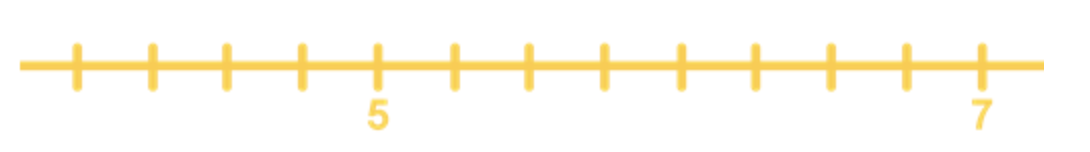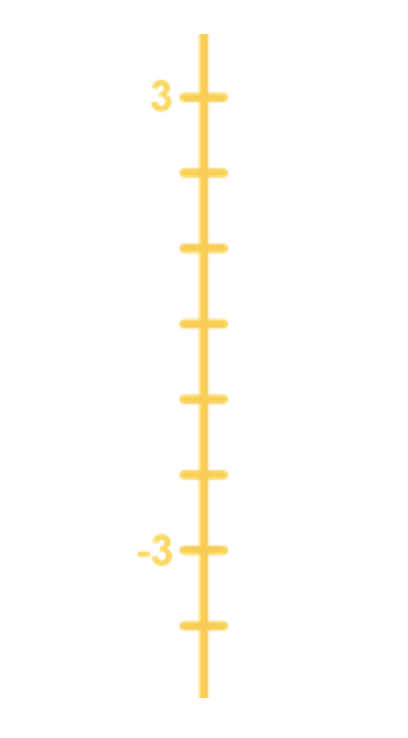# What is an integer#### Everything You Need in One Place

Homework problems? Exam preparation? Trying to grasp a concept or just brushing up the basics? Our extensive help & practice library have got you covered.#### Learn and Practice With Ease

Our proven video lessons ease you through problems quickly, and you get tonnes of friendly practice on questions that trip students up on tests and finals.#### Instant and Unlimited Help

Our personalized learning platform enables you to instantly find the exact walkthrough to your specific type of question. Activate unlimited help now!

##### Intros
###### Lessons
1. Introduction to What Is an Integer?
2. Definition of an Integer
3. Integers on a number line
4. Inequality symbols
##### Examples
###### Lessons
1. Representation of Integers

Find an integer for each of the following scenarios.

1. Tony bought a dozen of eggs.
2. Sabrina dropped 8 dollars.
3. Number of students in a class remains unchanged.
2. Understanding Integers

True or False.

1. On the negative region of the horizontal number line, as we move to the left, the numbers increase in value.
2. -8 > 2
3. The integers 5 and -5 are equally as far away from 0 on the number line.
3. Locating Integers on a Number Line

Fill in the missing numbers for each of the following number lines.

1.2.3.4. Comparing Integers Using Inequality Symbols

For each pair of integers below:

i. Locate them on a number line

ii. Write the correct inequality symbol (< or >) in each box.

1. -8 $\Box$ 2
2. 0 $\Box$ 10
3. -5 $\Box$ -3
###### Topic Notes

In this lesson, we will learn:

• Understanding Integers
• Representation of Integers
• Locating Integers on a Number Line
• Comparing Integers Using Inequality Symbols

• Integers include all the whole numbers and their negative selves.
• Whole numbers: all natural numbers including 0.
• Natural numbers: 1, 2, 3, …
• Number line:
• Can be either vertical or horizontal.
• Values in between intervals must be constant (not necessarily 1).
• Numbers increase in value as we move up/right.
• Inequality symbols:
• >: greater than
• <: less than